# Tutorial: Analyzing a model

This Tutorial page shows you how to do various kinds of sensitivity analysis to see how changes to input assumptions affect the results.

Tutorial Video: Analyze a model (6 minutes)

We use the Rent vs. Buy Analysis model, a modified version of the model that you used in Tutorial: Open a model to browse and Tutorial: Reviewing a model.

Open the Rent vs. Buy Analysis model from More Example models -> Tutorial models, by double-clicking the file labeled Rent vs. Buy Analysis.ana.

## Examine and change an uncertain input

When an input is defined as a probability distribution, a button with the name of the distribution appears next to the input’s name. Clicking this button opens the Object Finder window, in which you can see details and change the distribution’s parameters or type of distribution.

Rate of inflation’s button says Normal, indicating that it is defined as a normal distribution.

1.  Click the Normal button next to the Rate of inflation.

The Object Finder window appears. It shows that Rate of inflation is defined as a normal distribution with a mean of 3.5 and a standard deviation of 1.3.

You will now modify the probability distribution that defines Rate of inflation. Rather than using the normal distribution, you will use the uniform distribution, and assume that inflation has an equal probability of being anywhere between 3% and 4% per year.

2. Press the Library pulldown menu and change to the Discrete Distributions library

3.  Scroll down the list of distributions and select Uniform

4.  Change the minimum to 3.

5.  Change the maximum to 4.

6.  Click OK to accept the change.

7.  Click the Calc button to compute the new comparison of the present value of buying to renting.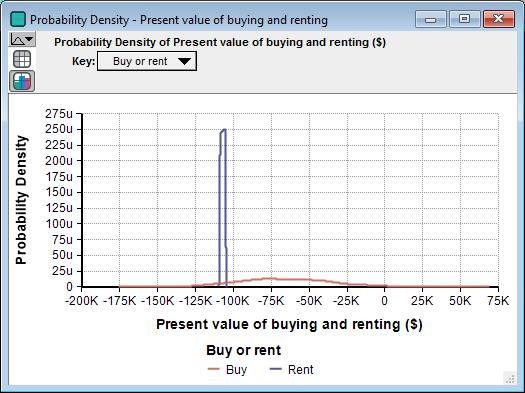The graphs show that the uncertainty in the cost of renting has narrowed to between about $105,000 and$109,000, while the uncertainty in the cost of buying has flattened to between about $125,000 and a gain of$10,000.

## Display alternative uncertainty views

Analytica offers a variety of views to display uncertain values, including selected statistics, probability bands, the probability density function, the cumulative probability distribution function, measures of central tendency, and the table of random numbers from which the uncertain distribution is estimated.

You will now examine several of these views.

In the upper-left corner of the Result window is the Uncertainty View popup menu.The miniature probability distribution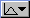indicates that Probability Density is selected.

The Result window now shows two cumulative probability curves. Along the vertical axis, these curves give the probability that each cost is less than a given value along the horizontal axis.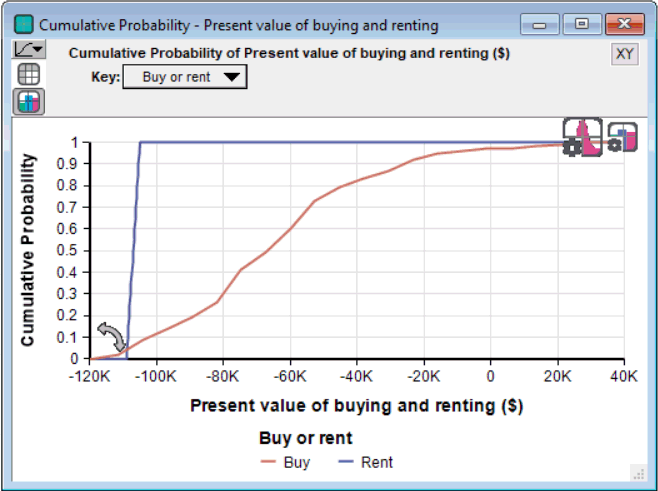There appears to be about a 50% probability that the cost to buy is below $70,000, while the cost to rent has a 50% probability of being below about$110,000.

Sometimes you might want to see an uncertain value expressed as a single number — a measure of central tendency. Analytica computes the mid value (sometimes called the deterministic value) by fixing all input probability distributions at their median (50% probability) values. The mid value is the only uncertainty view available for nonprobabilistic results.

The Result window now displays bar graphs for the two mid values.

Under the Uncertainty View popup menu are two buttons,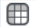and. Theis highlighted, indicating that the Result window is displaying a graph view. The Result window can also display numeric values in a spreadsheet-like table view.Analytica also provides the mean (or average) value.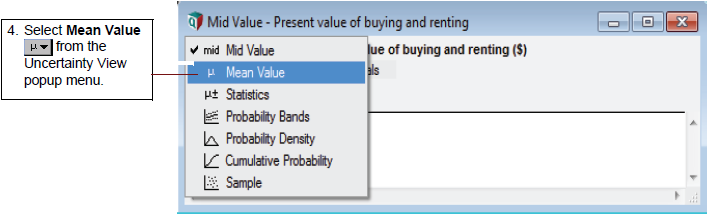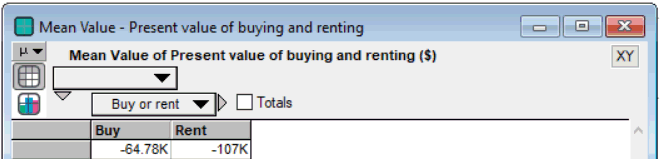You can also view a set of statistics, including both the median and mean, the ranges (minimum and maximum), and the standard deviation.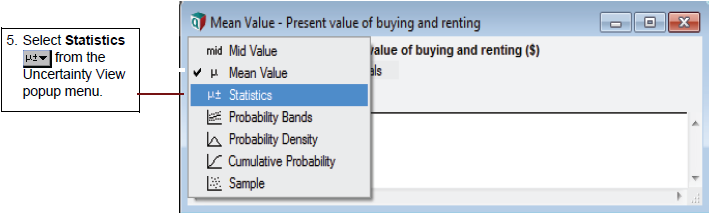The Result window now displays the minimum, median, mean, maximum, and standard deviation for Costs of buying and renting.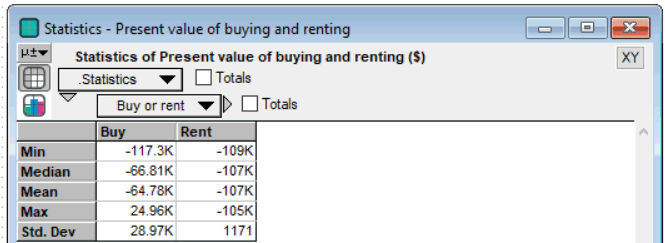The statistics might not be exact, because they are estimated from a sample of values from the distribution.

Finally, you see the sample values.The table above lists the 100 sample values that Analytica randomly generated from the probability distribution to estimate the statistics.

A sample size of 100 is adequate for most applications; however, if you need more precise estimates, you can increase the sample size. See Uncertainty Setup dialog in the Analytica User Guide.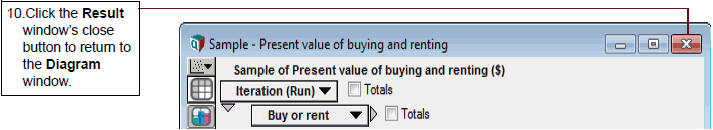## The difference between renting and buying

Next, we'll conduct importance analysis and parametric analysis in the model. Open the module called Model that you explored in Tutorial: Reviewing a model.

First look at the objective node, Difference between buying and renting.

1.  Click the Difference between buying and renting node to select it.

2.  Click the Result button to evaluate it.

The difference between the two uncertain values is uncertain. It is positive if it costs less to buy, and negative if it costs less rent over the time period.

## Importance analysis

Several of the input variables in the Rent vs. Buy Analysis model are uncertain, defined as probability distributions. It's useful to see how much of the uncertainty is in the result, in this case the Difference between buying and renting is due to each of these uncertain inputs. Importance analysis is an easy way to do this. Typically, a few key uncertain inputs are responsible for the lion’s share of the uncertainty in the output, while the rest of the inputs have little impact. If so, you can then focus your efforts on getting more precise estimates or building a more detailed model for those few most “important” inputs.

1.  Select the node Difference between buying and renting importance.

2.  Click the Result button to display importance values.

Importance is defined on a relative scale from 0 to 1. An importance value of 0 indicates that the uncertain input variable has no effect on the uncertainty in the output. A importance of 1 implies that you can "blame" all of the uncertainty in the output on that single input. Technically, it computes importance as the Rank correlation (also known as Spearman's correlation) between the output value and each uncertain input, using the RankCorrel function. In non-technical language, it is a relative measure for how much the uncertainty of an input contributes to the overall uncertainty of the output.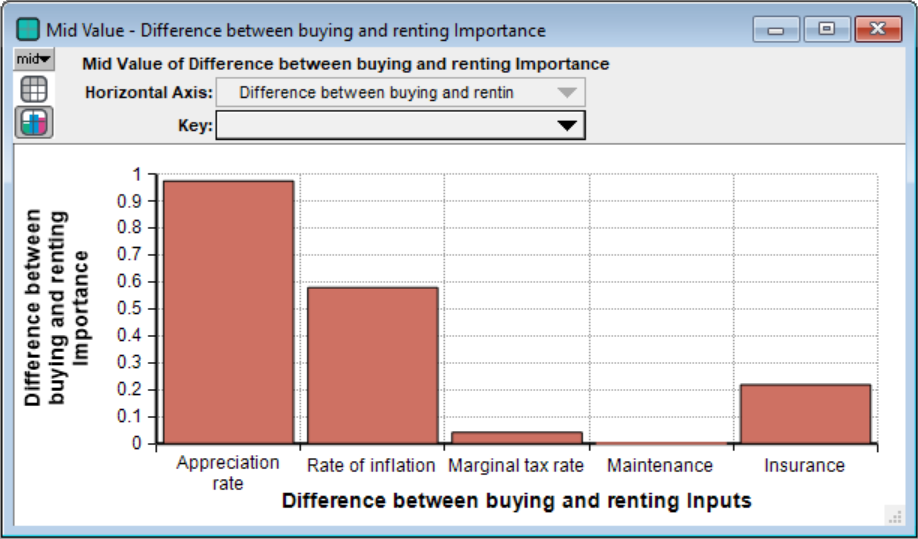We can see that the input Appreciation Rate contributes most of the uncertainty to the Difference between buying and renting.

For more information on how to create an importance variable in your own model see importance analysis and also Scatter plots in Statistics, Sensitivity, and Uncertainty Analysis.

## Parametric analysis

Parametric analysis involves varying the value of an input variable to examine its effect on a selected output. It helps you see how an input affects the desired outcome. Since the importance analysis you just did found that Appreciation rate caused most of the uncertainty in Difference between buying and renting, let's start the parametric analysis with that input variable. You want to change the Definition of Appreciation rate’ from a probability distribution to a list of alternative values.

1. Click the edit button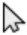in the toolbar to switch into edit mode. Then you can modify the model: adding and removing nodes, and modifying existing nodes.
2. Click the key icon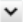to open the Attribute panel
3. Click the Appreciation rate node to select it
4. Select Definition from the Attribute dropdown menu to view its definition.
{{{3}}}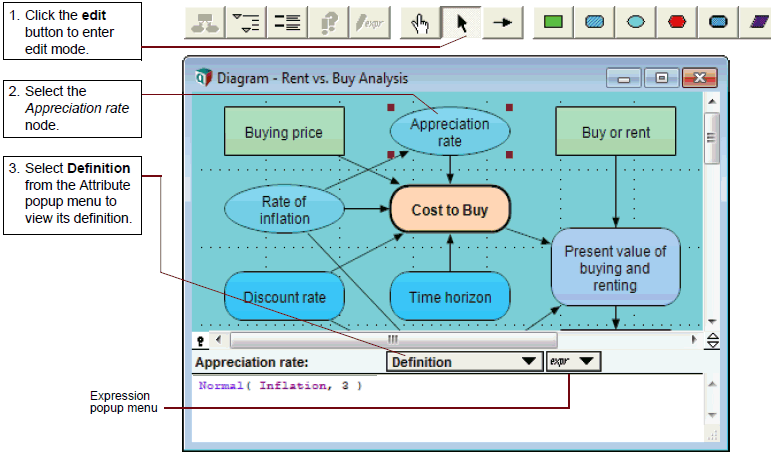When the Definition attribute is displayed, it shows the Expression popup menu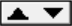, currently showing a probability distribution. You can press this to show a menu that lets you change the definition to any of these types of expression:

For now, you want to change the definition of Appreciation rate from a probability distribution to a list. Then you can enter a list of values from -10% to 10%.

4.  While pressing on the Expression popup menu, drag the mouse to List, and release the mouse button to select List.

5.  Click the OK button or press the Enter key to confirm that you want to change the definition from a distribution to a list.

Note that the icon on the Expression popup menu has changed to List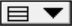.

After selecting a list, the definition shows a single cell ⁠— a box ⁠— containing the previous definition ⁠— in this case, a normal distribution Normal(Inflation,3)

Now replace it with a number, say -10:

6.  Select the cell by clicking in it. Type the value -10 and press the Tab key.

To add another cell to the list,

• Press the Enter key or down arrow key (not the numeric keypad Enter key). The next cell contains -9 (adding 1 to the previous value).
• Change its value to -5.
• Press Enter again, and it shows 0. The number in the new cell increments the previous cell by the difference between the last two cells, in this case by 5 ⁠— resulting in zero.
• Press Enter twice more, and it adds cells with 5 and 10. You can always override the automatic value by typing in something else.

7.  Type -5, followed by the Enter key. 0 automatically appears. Press the Enter key two more times; automatically, 5 and 10 appear.

8.  Select the Present value of buying and renting node.

9.  Select Mid Value from the Result menu.

Pivot the graph as follows:

10.  Select Appreciation rate for the Horizontal Axis.

11.  Select Buy or rent for the Key.

The resulting graph shows the mid value of buying and renting as Appreciation rate varies from -10% to 10%, using the list of values you just entered. You can see that renting and buying costs about the same at an Appreciation rate of -5% per year ⁠— the switchover value. It would be cheaper to rent if the Appreciation rate is less than -5%. And it would be cheaper to buy if it is greater than -5%.

12.  Click the Table button to view the result as a table.
{{{3}}}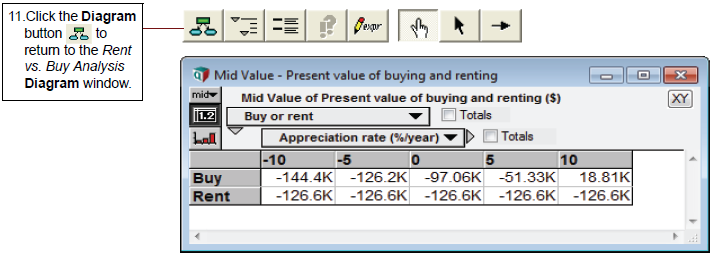The table shows the values computed for each parameterized value of Appreciation rate. Appreciation rate acts as an index ⁠— that is, a dimension of the table (array) of values in the Costs of buying and renting. You'll learn a lot more about indexes and arrays, a key source of Analytica's flexibility and power, in Tutorial: Arrays.

## Two-way parametric analysis

You can extend this parametric sensitivity analysis to two variables. In this cased, let's look at the effects of changing Buying price along with Appreciation rate.

1.  Select the Buying price node.

2. Select List from the Expression popup menu. Analytica asks you to confirm that you want to make this selection.

The first cell in this list contains the expression for the previous definition, 140K. Now change this value, and add additional cells, as you did for Appreciation rate.

4.  Click in the cell to select it. Type 120K and press the Enter key.

5. Type 140K and press the Enter key. 160K is automatically entered in the next cell.

6.  Select the Difference between buying and renting node.

7.  Select Mid Value from the Result menu to recompute and display its value.

The Result window displays the variable’s mid value. The Difference between buying and renting variable now shows three curves over Appreciation rate, one for each Buying price. The key shows the value of Buying price for each curve by color.

The graph shows that a $160K home, coupled with an appreciation rate of -2%/year or less, or a$140K home, coupled with an appreciation rate of -6%/year or less, results in renting being cheaper than buying. What is the best buy, a $120K home or a$160K home? That depends on the appreciation rate. If you expect an appreciation rate less than 9% per year, the less expensive home is the better investment. The more expensive home gives a larger return only for an appreciation rate over 9%.

{{{3}}}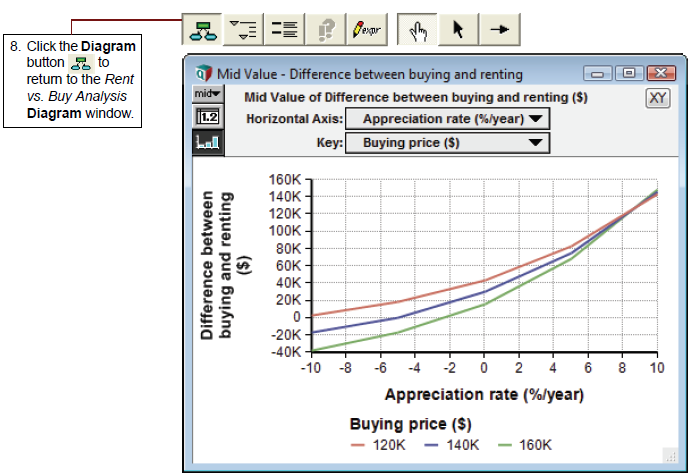### Three-way parametric analysis

So far the Cost of renting has been held constant. What happens if we change that too?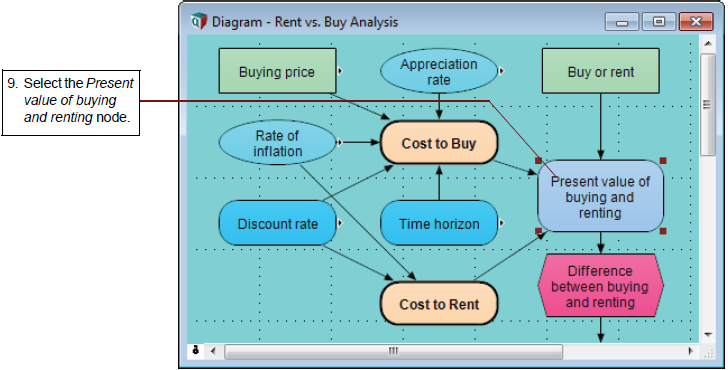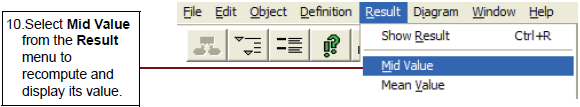Navigating arrows⠀

Navigating arrows⠀

Navigating arrows⠀

The result has now has three dimensions, Buying price, Buy or rent, and Appreciation rate. It shows two dimension in the graph, Appreciation rate along the X axis, and Buy or rent, in the Key. The third dimension Buying price is shown at the top of the graph as a slicer dimension, currently set to $120K. • Click the diagonal arrows to change the Buying price in the slicer index. 8. Click the down arrow to display a scrolling list of alternative values for the variable. The graph now show the mid value of Costs of buying and renting given that the Buying price equals$160K.

## Pivot a 3-dimensional graph or table

You can pivot the graph (or table) by selecting different variables to show in the X-axis, Key, or Slicer:

10.  Click the Table button to see the table view.

Buy or Rent becomes the third dimension with one value (buy) displayed.

This table shows the mid value cost of buying for the parameterized values of Buying Price and Appreciation Rate.

12.  Click the navigating arrow to show the corresponding table for Rent.

This table confirms that Cost to Rent does not vary with Buying Price or Appreciation rate ⁠— no surprise when you think about it!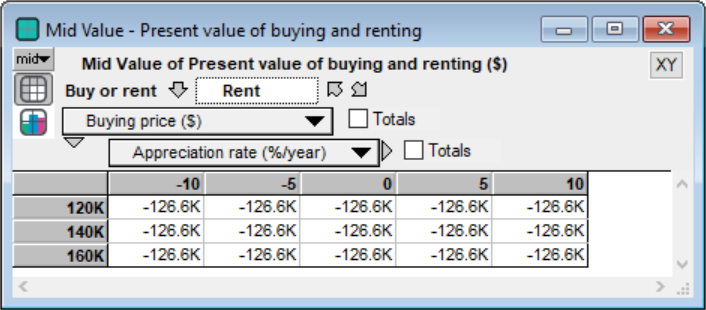You can quit Analytica at this point.

## Summary

This page showed several approaches to sensitivity analysis to find out how changes in key variables affect the results. The next page shows you how to create a new Analytica model.